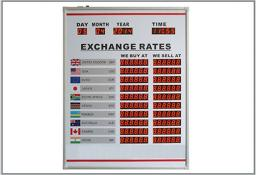# Exchanged 2214

In the table, the exchange rate list is an exchange office, but some values ​​are replaced by question marks. The exchange office exchanges money at the rates quoted and does not charge any other fees.

 nákup prodej 1 EUR 26,20 CZK 28,00 CZK 1 GBP b=? CZK c=? CZK

1. How many euros (a =?) Will the customer receive unless he exchanges CZK 4,200?

When a money changer buys £ 1,000 from a customer and then sells them all, his total profit is CZK 2,200. If, instead, the exchange sold 1,000 pounds and then exchanged all the torn crowns with another customer for pounds, he would earn 68.75 pounds.

2. For how many crowns does the exchange buy and sell 1 pound?

a =  150 Eur
b =  32 CZK
c =  34.2 CZK

### Step-by-step explanation:

a = 4200/28
1000 (c-b) = 2200
1000 (c-b) = 68.75 b

a = 4200/28
1000·(c-b) = 2200
1000·(c-b) = 68.75·b

28a = 4200
1000b-1000c = -2200
1068.75b-1000c = 0

Pivot: Row 2 ↔ Row 3
28a = 4200
1068.75b-1000c = 0
1000b-1000c = -2200

Row 3 - 1000/1068.75 · Row 2 → Row 3
28a = 4200
1068.75b-1000c = 0
-64.327c = -2200

c = -2200/-64.32748538 = 34.2
b = 0+1000c/1068.75 = 0+1000 · 34.2/1068.75 = 32
a = 4200/28 = 150

a = 150
b = 32
c = 171/5 = 34.2

Our linear equations calculator calculates it.Did you find an error or inaccuracy? Feel free to write us. Thank you!

Tips for related online calculators
Do you have a system of equations and looking for calculator system of linear equations?